# Physics of Welding Physics of Welding Lesson Objectives

• Slides: 28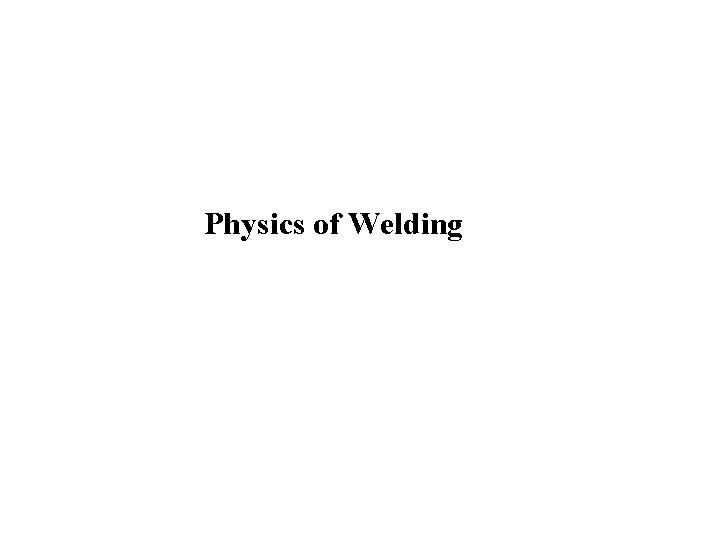Physics of WeldingPhysics of Welding Lesson Objectives When you finish this lesson you will understand: • Heat input and heat transfer from arc to weld • Metal melting and regions of weld. • Arc physics and plasma properties of arc Learning Activities 1. Read Handbook pp 32 -62 2. Look up Keywords 3. View Slides; 4. Read Notes, 5. Listen to lecture 6. Do on-line workbook 7. Do homework Keywords: Heat Input, Heat Transfer Efficiency, Heat Affected Zone, Enthalpy of Melting, Latent Heat, Melting efficiency, Plasma, Polarity, Thermionic Work Function, Ionization, Cathode Spot, Anode Spot, Arc I-V Characteristics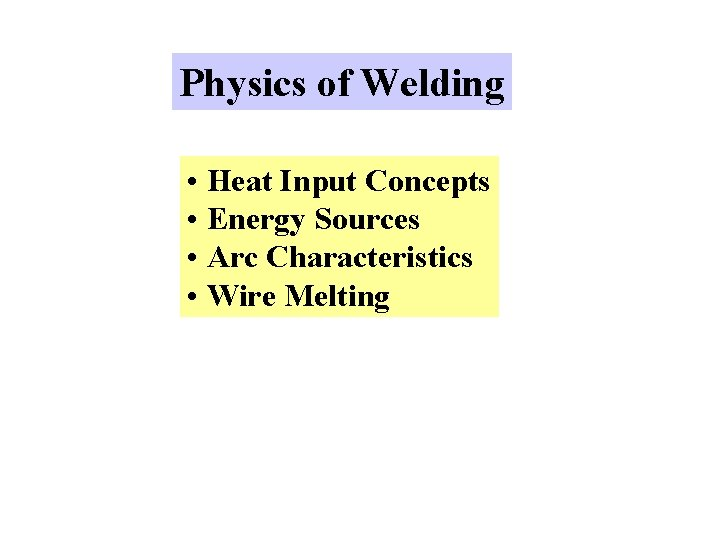Physics of Welding • Heat Input Concepts • Energy Sources • Arc Characteristics • Wire MeltingHeat Loss Heat input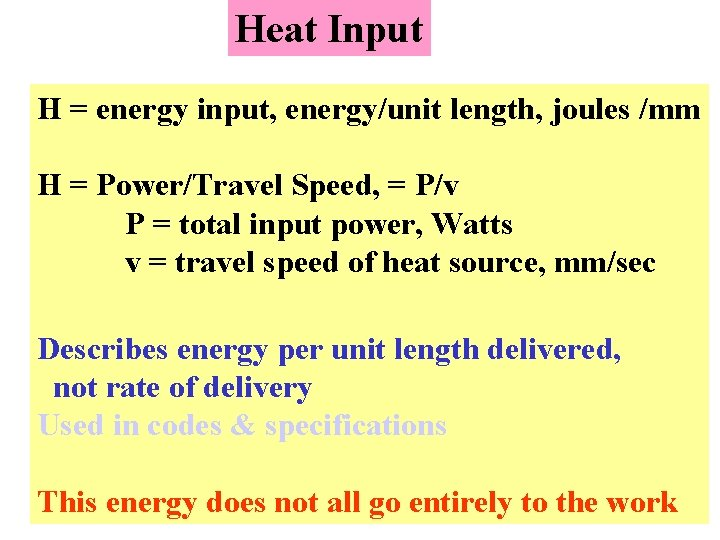Heat Input H = energy input, energy/unit length, joules /mm H = Power/Travel Speed, = P/v P = total input power, Watts v = travel speed of heat source, mm/sec Describes energy per unit length delivered, not rate of delivery Used in codes & specifications This energy does not all go entirely to the workHeat Input for Arcs H = P/v = EI/v E = Arc Voltage (Volts) I = Arc Current (Amps) EI = Process power, converted to Heat v = Welding Travel Speed Not all the arc energy goes into the work Hnet = f 1 H = f 1 P/v = f 1 EI/v f 1 = Heat Transfer Efficiencyf 1 = Heat Transfer Efficiency short Arc Length longMelted Base Metal Reinforcement Heat Affected Zone Aw = Cross Section of Weld = Am + Ar For Autogenous Weld (no filler metal) Aw = A m Q= Heat Required to melt a Given Volume of Weld = Heat Required to elevate solid to MP + Latent Heat of Fusion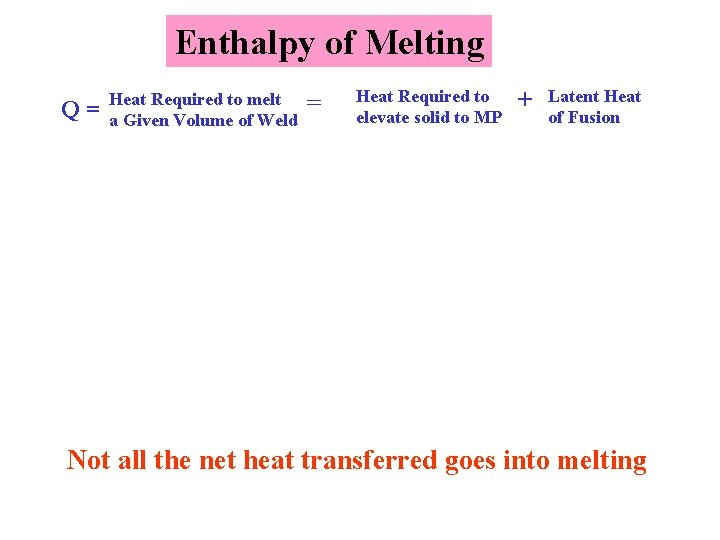Enthalpy of Melting Q= Heat Required to melt a Given Volume of Weld = Heat Required to elevate solid to MP + Latent Heat of Fusion Not all the net heat transferred goes into meltingMelting Efficiency f 2 = Melting efficiency, the fraction of the process heat energy per unit length delivered to the metal which is required to melt the metal f 2 = QAw/Hnet f 2 = QAwv/f 1 EI From previous slide: Hnet = f 1 H = f 1 P/v = f 1 EI/v Melting Efficiency Depends On: • Higher Thermal Conductivity - Lower Efficiency • High Energy Density Heat Source - Higher Efficiency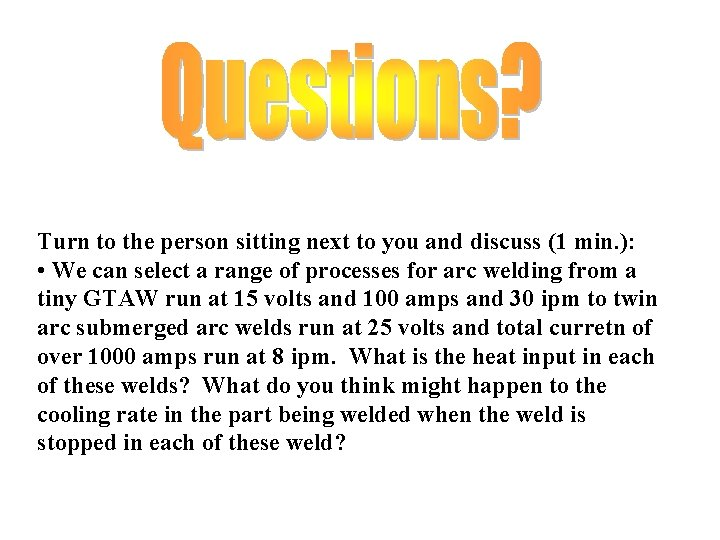Turn to the person sitting next to you and discuss (1 min. ): • We can select a range of processes for arc welding from a tiny GTAW run at 15 volts and 100 amps and 30 ipm to twin arc submerged arc welds run at 25 volts and total curretn of over 1000 amps run at 8 ipm. What is the heat input in each of these welds? What do you think might happen to the cooling rate in the part being welded when the weld is stopped in each of these weld?Other Energy Sources Arc H = EI/v Resistance: H = I 2 Rt Electroslag: H = EIt Laser: H = Heat generated, joules E = Voltage, volts v = Travel Speed, mm/sec I = Current, amps R = Resistance, ohms t = Time, sec PD = Power Density P 1 = Input power EB: A = Area of focused beamOXYFUEL GAS WELDING THERMIT WELDING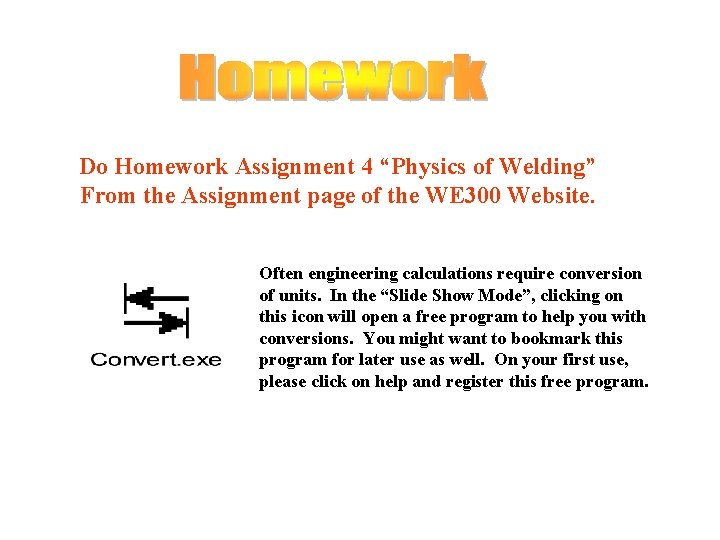Do Homework Assignment 4 “Physics of Welding” From the Assignment page of the WE 300 Website. Often engineering calculations require conversion of units. In the “Slide Show Mode”, clicking on this icon will open a free program to help you with conversions. You might want to bookmark this program for later use as well. On your first use, please click on help and register this free program.Polarity and Current Flow Welding Electrode or "Electrode" Anode Cathode I DCEP I DCEN Cathode Anode Work Electrode or "Work" Reverse RPEP Straight SPEN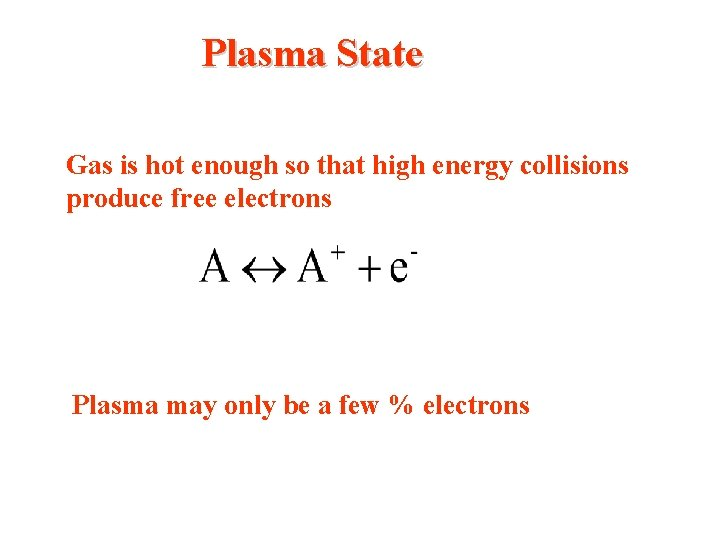Plasma State Gas is hot enough so that high energy collisions produce free electrons Plasma may only be a few % electronsConduction of Current in the Arc Cathode Thermal Ionization Electrons Emitted Free Electron Ion Plasma T>10, 000 K Anode Recombination Neutral Gas Atom Electrons Absorbed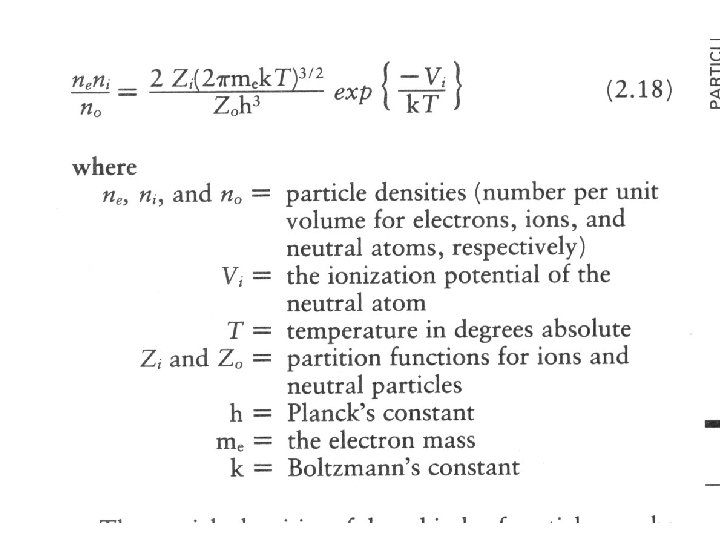Argon ArcThermionic Work Function Energy Required for electron to escape a solid surface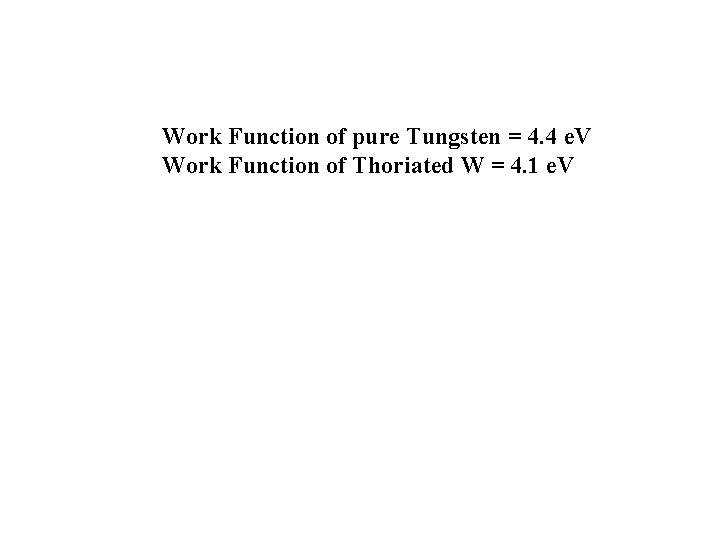Work Function of pure Tungsten = 4. 4 e. V Work Function of Thoriated W = 4. 1 e. V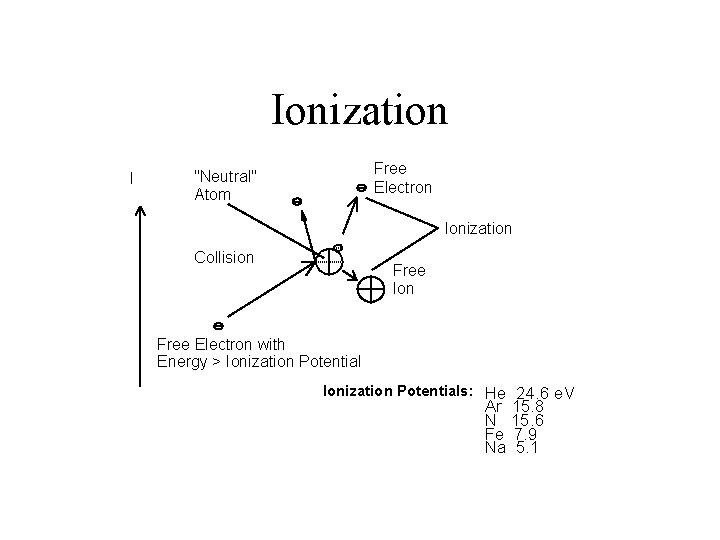Ionization I Free Electron "Neutral" Atom Ionization Collision Free Ion Free Electron with Energy > Ionization Potentials: He 24. 6 e. V Ar 15. 8 N 15. 6 Fe 7. 9 Na 5. 1} } } Will total voltage change if we change the amount of current (say from 200 amps to 300 amps)?Arc V-I Characteristic Unstable We see that current and arc length have an effect, what happens if we change from Ar to some other ionizing gas?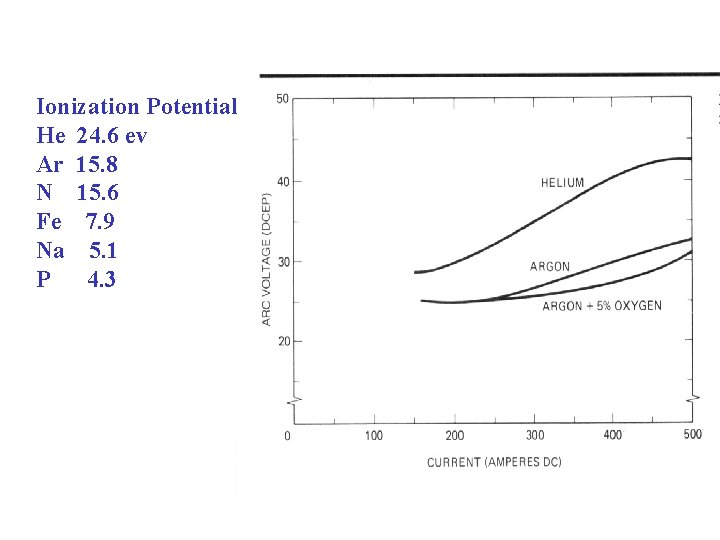Ionization Potential He 24. 6 ev Ar 15. 8 N 15. 6 Fe 7. 9 Na 5. 1 P 4. 3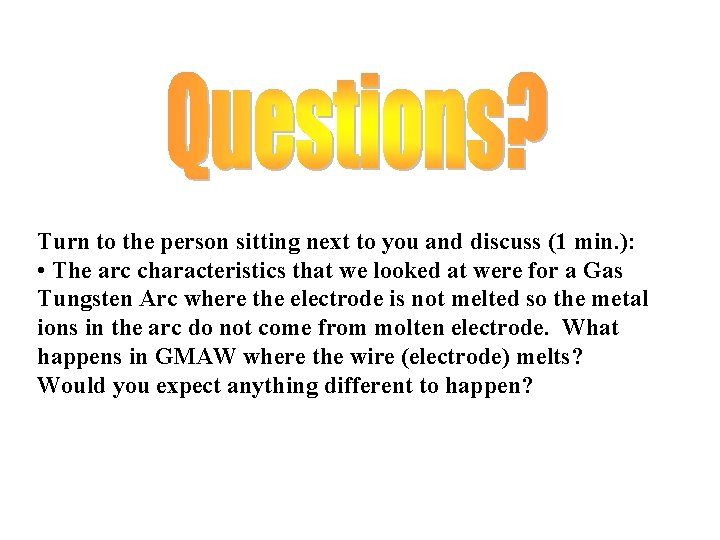Turn to the person sitting next to you and discuss (1 min. ): • The arc characteristics that we looked at were for a Gas Tungsten Arc where the electrode is not melted so the metal ions in the arc do not come from molten electrode. What happens in GMAW where the wire (electrode) melts? Would you expect anything different to happen?